# ComplexHeatmap: Create Complex Heatmaps in R

Heatmap is a statistical visualization method for visualizing complex data sets in matrix form and quickly gaining insights from large datasets.

Heatmaps are widely used in bioinformatics for analyzing and visualizing large gene expression datasets obtained from different samples and conditions.

This tutorial explains how to use the `Heatmap()` function from the `ComplexHeatmap` R Bioconductor package for visualizing complex heatmaps.

## Install ComplexHeatmap

You can install the `ComplexHeatmap` R package (from Bioconductor) as below:

``````if (!require("BiocManager", quietly = TRUE))
install.packages("BiocManager")

BiocManager::install("ComplexHeatmap")
``````

## Getting the dataset

We will use the sample RNA-seq gene expression dataset for creating heatmaps using the `ComplexHeatmap` package.

``````# load dataset
# convert to matrix
df_mat = data.matrix(df)
# view first few rows of data
# output
A         B         C        D        E         F
B-CHI1 4.505700  3.260360 -1.249400  8.89807  8.05955 -0.842803
CTL2-1 3.508560  1.660790 -1.856680 -2.57336 -1.37370  1.196000
B-CHI2 2.160030  3.146520  0.982809  9.02430  6.05832 -2.967420
CTL2-2 1.884750  2.295690  0.408891 -3.91404 -2.28049  1.628820
CHIV1  0.255193 -0.761204 -1.022350  3.65059  2.46525 -1.188140
``````

## Basic heatmaps

Create and visualize a single heatmap with the default settings,

``````# load package
library(ComplexHeatmap)

# visualize heatmap
Heatmap(df_mat)
``````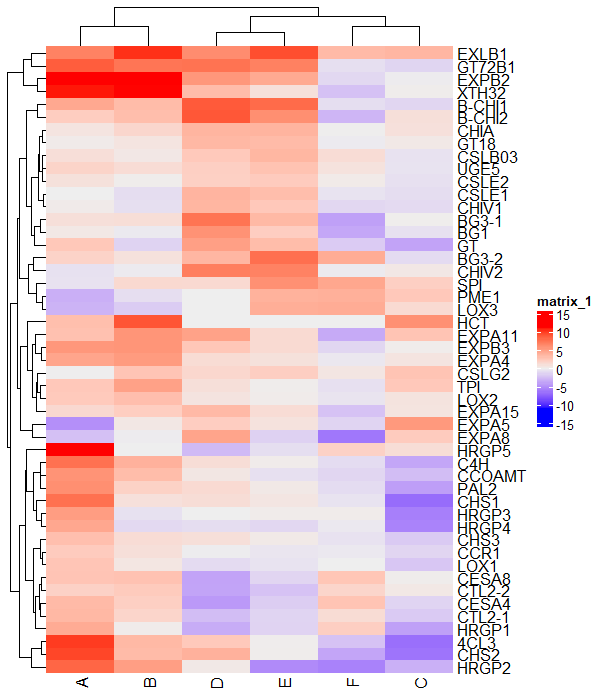## Change color of the heatmap

You can change the color of the heatmap using the `col` argument

``````# create color scale
library(circlize)
col_fun = colorRamp2(seq(min(df_mat), max(df_mat), length = 3),
c("green", "black", "red"))

# visualize heatmap
Heatmap(df_mat, col = col_fun)
``````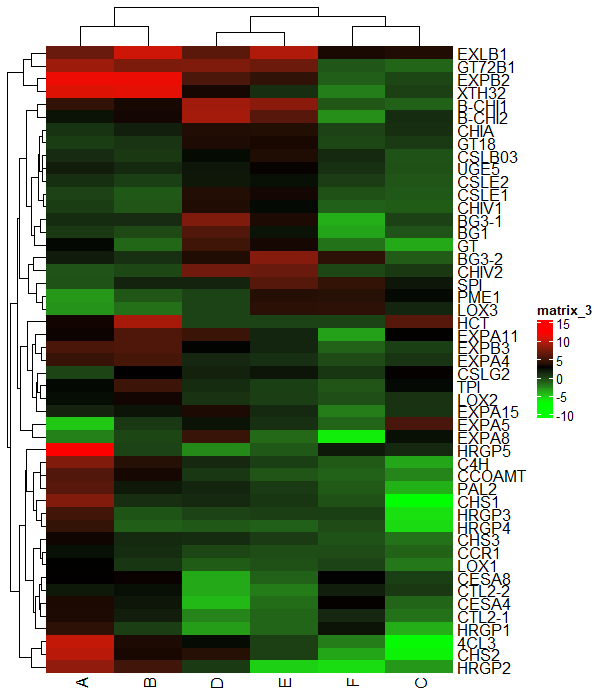## Change borders of the heatmap

You can change the individual cell borders of the heatmap using the `rect_gp` argument

``````Heatmap(df_mat, rect_gp = gpar(col = "white", lwd = 2))
``````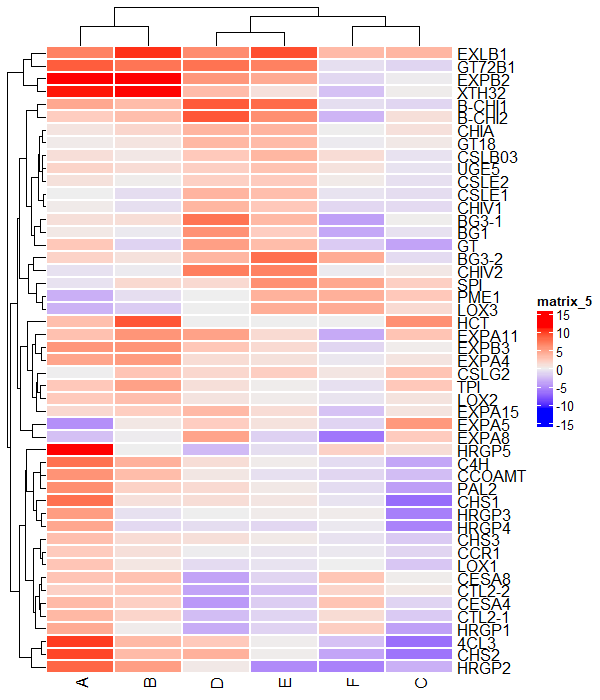## Add titles to the heatmap

You can add row and column titles to the heatmap using the `column_title` and `row_title` arguments

``````Heatmap(df_mat, column_title = "Conditions", row_title = "Genes",
column_title_side = "bottom")
``````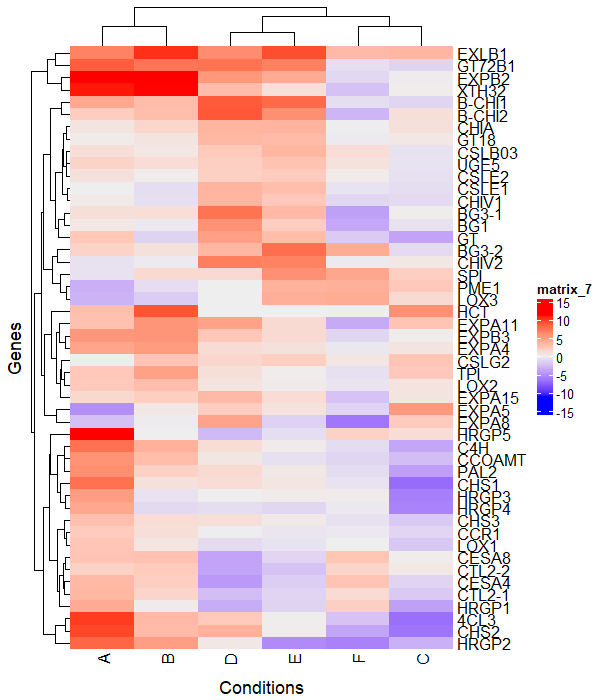### Clustering

The row and column clustering is plotted by default in ComplexHeatmap.

You can turn off row and column clustering using `cluster_rows` and `show_column_dend` arguments

``````# turn off row clustering
Heatmap(df_mat, cluster_rows = FALSE)

# turn off column clustering
Heatmap(df_mat, show_column_dend = FALSE)
``````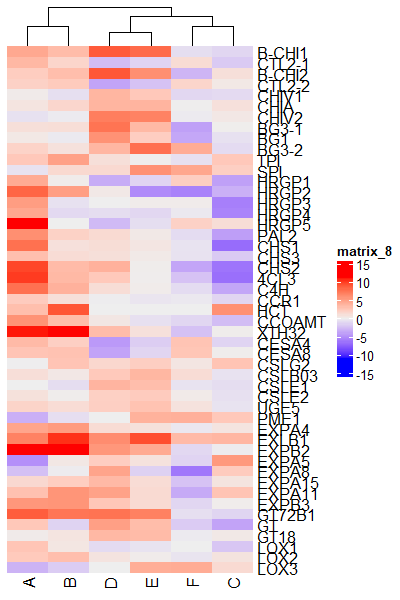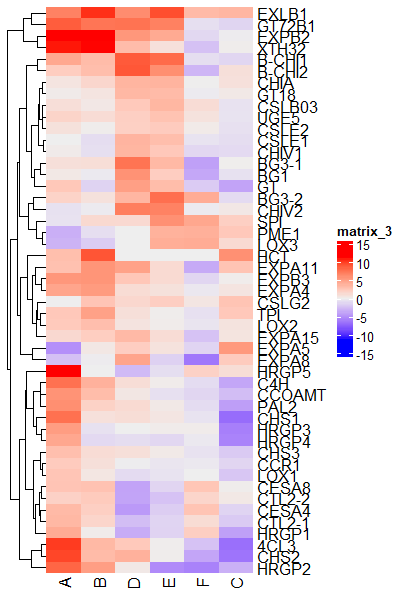### Color the clusters

You can also color the individual row clusters,

``````# install.packages("dendextend")
library(dendextend)
row_dend = as.dendrogram(hclust(dist(df_mat)))

# color row clustering
Heatmap(df_mat, cluster_rows = color_branches(row_dend, k = 5))
``````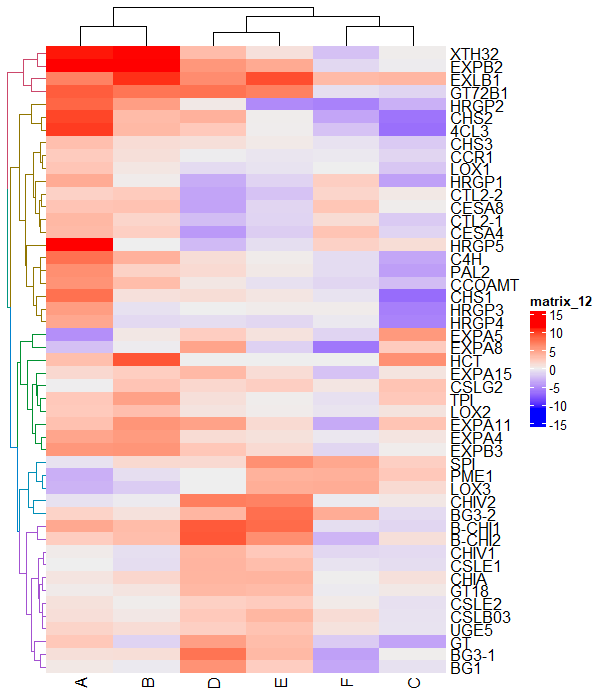### Split the heatmaps

You can also split the heatmap by rows and columns to better understand the clustering of the data. It uses k-means clustering to split the clusters.

``````# split row clusters
Heatmap(df_mat, name = "scale", row_km = 5)

# split column clusters
Heatmap(df_mat, name = "scale", column_km = 2)
``````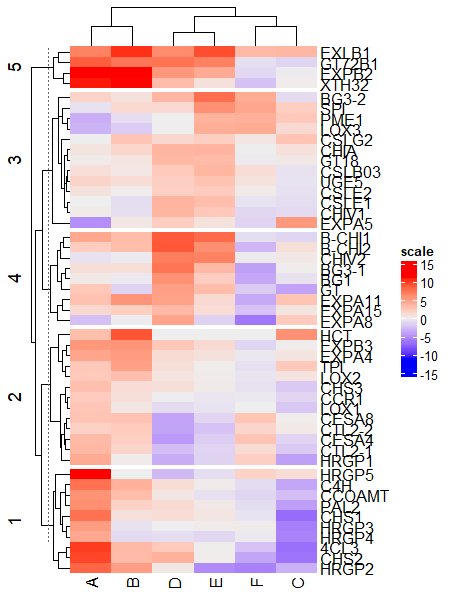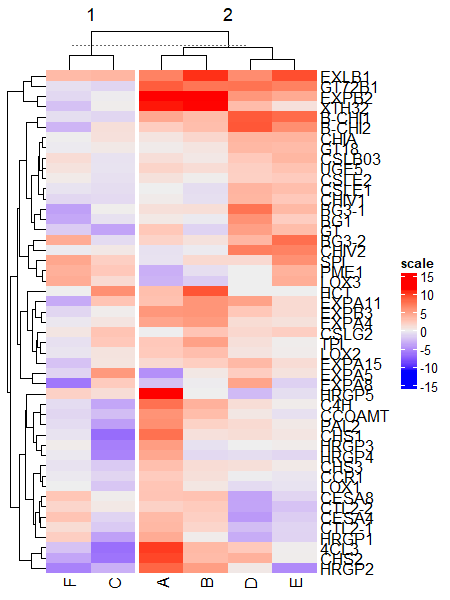Split by both rows and columns simultaneously,

``````# split row and column clusters at same time
Heatmap(df_mat, name = "scale", row_km = 5, column_km = 2)

``````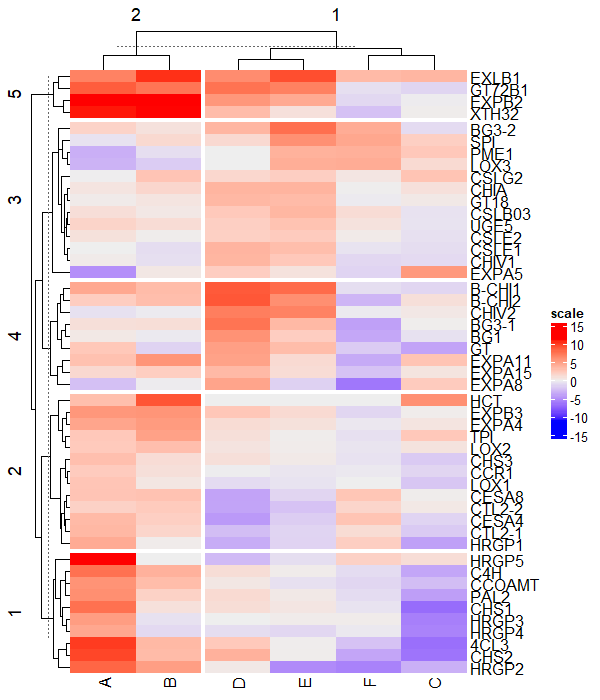### Change legend position

You can change the legend position in the ComplexHeatmap as below,

``````draw(Heatmap(df_mat), heatmap_legend_side = "left")
``````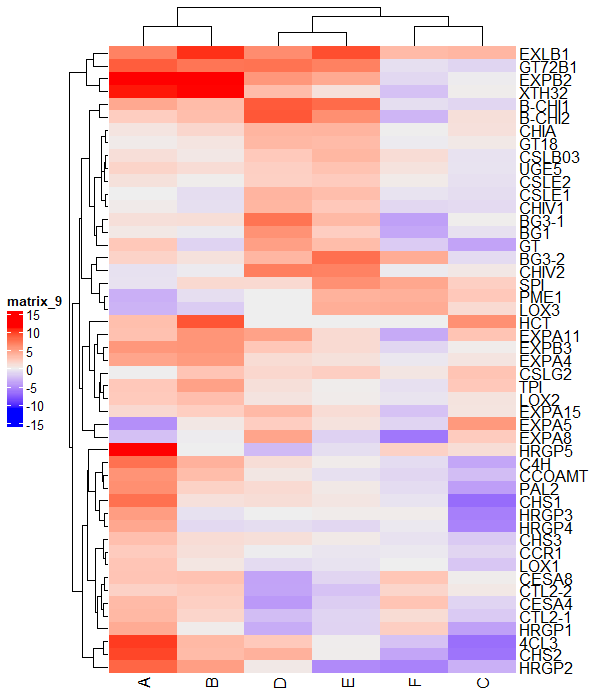Similarly, you can use the `bottom` and `top` positions to adjust the legend position.

## Subscribe to get new article to your email when published

* indicates required